# What Is An Equation With Two Solutions? (3 Key Ideas)

In mathematics, we want to solve an equation to find the values of variables that make it true.  However, there are some cases when an equation has more than one solution.

So, what is an equation with two solutions?  An equation with two solutions is one that is true for two different values of a variable. We can have absolute value equations, radical equations, and quadratic equations with two solutions.

Of course, there may be more than one way to show that an equation has two solutions.  For example, in some cases we can use algebra to factor, use the quadratic formula, or draw a graph to show that two solutions exist.

In this article, we’ll talk about various equations with two solutions.  We’ll also look at some examples to make the concept clear.

Let’s get started.

## What Is An Equation With Two Solutions?

An equation with two solutions is one that is true for exactly two values of a variable.  Keep in mind that these solutions can be real or complex.

For example, a quadratic equation with two solutions can have two real solutions or two complex conjugate solutions.  There are two cases for the graph:

• In the case of two real solutions, the graph of the quadratic (a parabola) will intersect the x-axis twice (at the zeros).
• In the case of two complex solutions, the graph of the quadratic (a parabola) will not intersect the x-axis at all (since there are no real zeros).

We can also construct an equation with two solutions by choosing our two solutions in advance.  For example, if we want a quadratic equation with solutions x = r and x = s, we just FOIL the following factors:

• f (x) = a(x – r)(x – s)
• =ax2 – a(r + s)x + ars

where a is any nonzero value.

For example, let’s say we want to construct a quadratic function with zeros at x = 5 and x = -3.  Then, using the formula above, we find:

• f (x) = a(x – 5)(x – (-3))
• =a(x – 5)(x + 3)
• =a(x2 – 2x – 15)

Let’s say we want the parabola to go through the point (2, 30).  We can solve for a with x = 2 and f(2) = 30:

• f(x) = a(x2 – 2x – 15)
• f(2) = a(22 – 2(2) – 15)
• 30 = a(4 – 4 – 15)
• 30 = -15a
• -2 = a

So, with a = -2, we get a quadratic function with solutions x = 5 and x = -3 that passes through the point (2, 30):

• f(x) = a(x2 – 2x – 15)
• f(x) = -2(x2 – 2x – 15)
• f(x) = -2x2 +4x + 30

You can see the graph of the corresponding parabola below.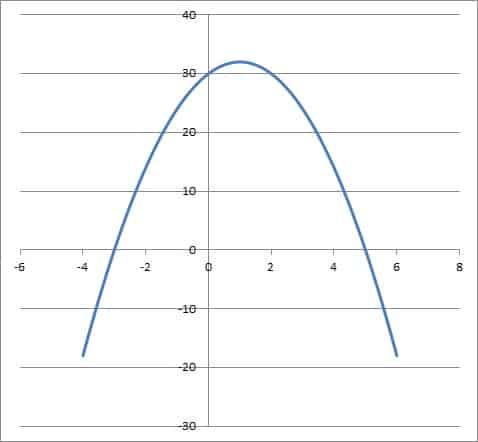The graph of the parabola f(x) = -2x2 +4x + 30 has zeros at x = -3 and x = 5. It also passes through the point (2, 30).

We can find a few different types of equations with two solutions, including:

• Absolute Value Equations With Two Solutions
• Radical Equations With Two Solutions
• Quadratic Equations With Two Solutions

Let’s take a look at each of these situations in turn, along with some examples to illustrate the idea.  We’ll start with absolute value equations with two solutions.

## Absolute Value Equations With Two Solutions

An absolute value equation can have two solutions if the absolute value is equal to a positive number.  This is because absolute value is a distance metric (it measures the distance from a value to zero on the number line).

Since there are two values that are a distance of D from zero (both D and –D), there are two solutions to the absolute value equation |x| = D.

Remember that an absolute value cannot be negative, but the solutions can be.  (If you need a refresher, check out my article here.)

If we graph both sides of an absolute value equation with two solutions, there will be two points of intersection (these correspond to the two solutions).

Let’s look at some examples.

### Example 1: Absolute Value Equation With Two Solutions

Consider the absolute value equation

• |2x – 4| = 6

We can already tell that there are two solutions, since the absolute value on the left side is equal to a positive number on the right.  So, we need to look at two separate “halves” to solve the equation:

First, we solve:

• 2x – 4 = 6
• 2x = 6 + 4
• 2x = 10
• x = 5

Then, we solve:

• 2x – 4 = -6
• 2x = -6 + 4
• 2x = -2
• x = -1

So, the two solutions to this absolute value equation are x = -1 and x = 5.  We can confirm both solutions by substituting back into the original absolute value equation.

You can see from the graph below that the two functions y = |2x – 4| and y = 6 intersect at x = -1 and x = 5 (the y-values are 6 for both points).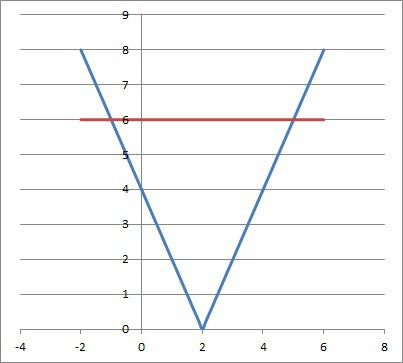The graph of y = |2x – 4| (blue) intersects the horizontal line y = 6 (red) at x = -1 and x = 5

### Example 2: Absolute Value Equation With Two Solutions

Consider the absolute value equation

• |-3x – 6| = 27

We can already tell that there are two solutions, since the absolute value on the left side is equal to a positive number on the right.  So, we need to look at two separate “halves” to solve the equation:

First, we solve:

• -3x – 6 = 27
• -3x = 27 + 6
• -3x = 33
• x = -11

Then, we solve:

• -3x – 6 = -27
• -3x = -27 + 6
• 3x = -21
• x = 7

So, the two solutions to this absolute value equation are x = 7 and x = -11.  We can confirm both solutions by substituting back into the original absolute value equation.

You can see from the graph below that the two functions y = |-3x – 6| and y = 27 intersect at x = 7 and x = -11 (the y-values are 27 for both points).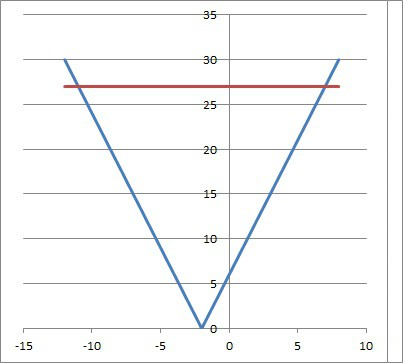The graph of y = |-3x – 6| (blue) intersects the horizontal line y = 27 (red) at x = 7 and x = -11.

## Radical Equations With Two Solutions

A radical equation can have two solutions if there is a squared term under a radical with index 2 (a square root).  This is because there will be two values of the variable that can yield a solution.

Let’s look at some examples.

### Example 1: Radical Equation With Two Solutions

• √(x2 + 6x + 9) = 5

Since the radical (a square root) has an index of 2, the approach for solving is to square both sides (raise both sides to the power of 2):

• √(x2 + 6x + 9) = 5
•  (√(x2 + 6x + 9))2 = (5)2  [square both sides]
• x2 + 6x + 9 = 25
• x2 + 6x – 16 = 0  [subtract 9 from both sides]
• (x + 8)(x – 2) = 0  [factor]

So x = -8 and x = 2 are the solutions of this equation.  We can plug both of these values back into the original radical equation to check them.

You can see from the graph below that the two curves y = √(x2 + 6x + 9) and y = 5 intersect at x = -8 and x = 2 (the y-values are 5 for both points).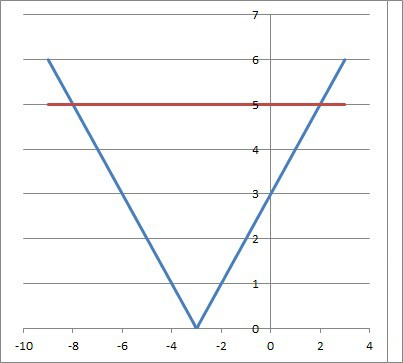The graph of √(x2 + 6x + 9) (blue) intersects the horizontal line y = 5 (red) at x = -8 and x = 2.

### Example 2: Radical Equation With Two Solutions

• √(x2 – 8x + 16) = 6

Since the radical (a square root) has an index of 2, the approach for solving is to square both sides (raise both sides to the power of 2):

• √(x2 – 8x + 16) = 6
•  (√(x2 – 8x + 16))2 = (6)2  [square both sides]
• x2 – 8x + 16 = 36
• x2 – 8x – 20 = 0  [subtract 9 from both sides]
• (x – 10)(x + 2) = 0  [factor]

So x = 10 and x = -2 are the solutions of this equation.  We can plug both of these values back into the original radical equation to check them.

You can see from the graph below that the two curves y = √(x2 – 8x + 16)and y = 6 intersect at x = 10 and x = -2 (the y-values are 6 for both points).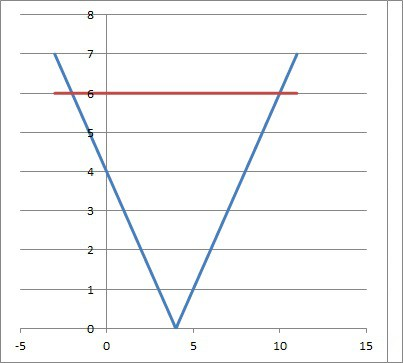The graph of √(x2 – 8x + 16) (blue) intersects the horizontal line y = 6 (red) at x = 10 and x = -2.

## Quadratic Equations With Two Solutions

A quadratic equation in standard form has two solutions it the discriminant is nonzero.  That is, if b2 – 4ac is not zero, or b2 is not equal to 4ac.

This presents two cases:

• Case 1: The discriminant is positive (b2– 4ac > 0 or b2 > 4ac).  In this case, there are two real solutions to the quadratic equation.
• Case 2: The discriminant is negative (b2 – 4ac < 0 or b2 < 4ac).  In this case, there are two complex conjugate solutions to the quadratic equation.

The second case may occur if we try to find the intersection of a parabola and a line that never meet.

Let’s look at some examples.

### Example 1: Quadratic Equation With Two Real Solutions

• 2x2 – 14x + 24 = 0

We can divide by 2 on both sides to simplify and get smaller coefficients:

• x2 – 7x + 12 = 0

This is a quadratic in standard form with a = 1, b = -7, and c = 12.  This gives us a discriminant of:

• b2 – 4ac
• =(-7)2 – 4(1)(12)
• =49 – 48
• =1

So the discriminant is 1, which is positive.  This means the quadratic equation has two real solutions.

We can find the exact solutions by using the quadratic formula, or we can factor easily to get:

• x2 – 7x + 12 = 0
• (x – 3)(x – 4) = 0

So our solutions are x = 3 and x = 4.

You can see from the graph below that the parabola y = 2x2 – 14x + 24intersects the x-axis at x = 3 and x = 4.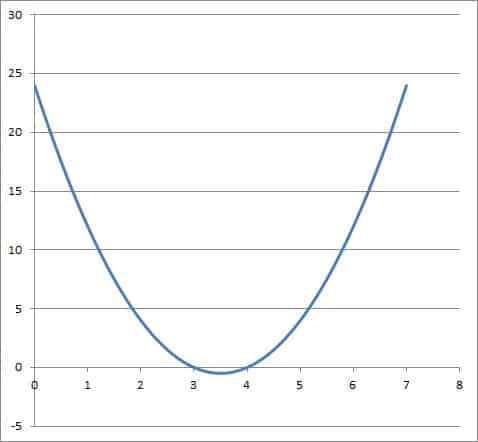The graph of the parabola y = 2x2 – 14x + 24 intersects the x-axis at x = 3 and x = 4

### Example 2: Quadratic Equation With Two Complex Conjugate Solutions

Let’s say we want to find out where the following two shapes intersect:

• The line f(x) = 3x + 6
• The parabola g(x) = 5x2 – x + 7

To find the points of intersection, we set the two functions equal and solve:

• f(x) = g(x)  [set line and parabola equal]
• 3x + 6 = 5x2 – x + 7  [definitions of f(x) and g(x)]
• 0 = 5x2 – 4x + 1

This is a quadratic in standard form with a = 5, b = -4, and c = 1.  This gives us a discriminant of:

• b2 – 4ac
• =(-4)2 – 4(5)(1)
• =16 – 20
• =-4

So the discriminant is -4, which is negative.  This means the quadratic equation has two complex conjugate solutions.

We can find the exact solutions by using the quadratic formula to get: x = (2 + i) / 5 and x = (2 – i) / 5.

You can see from the graph below that the parabola f(x) = 5x2 – x + 7 never intersects the line f(x) = 3x + 6.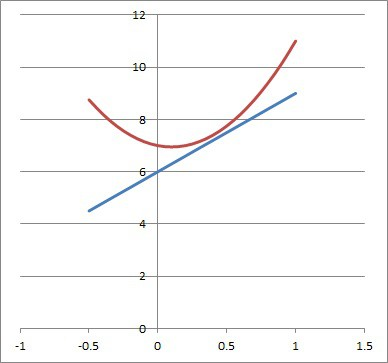The parabola f(x) = 5x2 – x + 7 (red) never intersects the line f(x) = 3x + 6 (blue).

## Conclusion

Now you know about some scenarios where you might see an equation with two solutions.  You also know what to look for to tell that there are two solutions to an equation.

You might also want to read my article on equations with no solution.